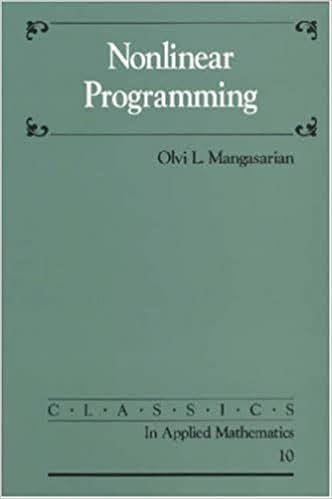# Classics in Applied Mathematics Volume 10 - Nonlinear Programming

Discussion in 'Reference Textbooks' started by vietnixvn, Apr 26, 2021.

1.This reprint of the 1969 book of the same name is a concise, rigorous, yet accessible, account of the fundamentals of constrained optimization theory. Many problems arising in diverse fields such as machine learning, medicine, chemical engineering, structural design, and airline scheduling can be reduced to a constrained optimization problem. This book provides readers with the fundamentals needed to study and solve such problems. Beginning with a chapter on linear inequalities and theorems of the alternative, basics of convex sets and separation theorems are then derived based on these theorems. This is followed by a chapter on convex functions that includes theorems of the alternative for such functions. These results are used in obtaining the saddlepoint optimality conditions of nonlinear programming without differentiability assumptions.
• ASIN : 0898713412
• Publisher : Society for Industrial and Applied Mathematics (January 1, 1987)
• Language : English
• Paperback : 236 pages
• ISBN-10 : 9780898713411
• ISBN-13 : 978-0898713411
• Item Weight : 11.8 ounces
• Dimensions : 6.25 x 0.5 x 9.25 inches